# Bihar Board Class 10 Science Solutions Chapter 1 Chemical Reactions and Equations

## BSEB Bihar Board Class 10 Science Solutions Chapter 1 Chemical Reactions and Equations

Bihar Board Class 10 Science Solutions Chapter 1 Chemical Reactions and Equations Textbook Questions and Answers, Additional Important Questions, Notes.

### Bihar Board Class 10 Science Chapter 1 Chemical Reactions and Equations InText Questions and Answers

In-text Questions (Page 6)

Question 1.
Why should magnesium ribbon be cleaned before v burning in air ?
Magnesium ribbon should be cleaned to remove the dust particles present at the upper surface and so that the surface comes directly in contact with air.

Question 2.
Write the balanced equation for the following chemical reactions :

1. Hydrogen + chlorine → Hydrogen chloride
2. Sodium + water → Sodium hydroxide + hydrogen

1. H2 + Cl2 → 2HCl
2. 2Na + 2H2O → 2NaOH + H2

Question 3.
Write a balanced chemical equation with state symbols for the following reactions :

1. Solution of barium chloride and sodium sulphate in water to give insoluble barium sulphate and the solution of sodium chloride.
2. Sodium hydroxide solution (in water) reacts with hydrochloric acid solution (in water) to produce sodium chloride solution and water.

1. BaCl2(l) + Na2SO4(aq) → BaSO4(s) + 2NaCl(l)
2. NaOH(aq) + HCl(aq) → NaCl(l) + H2O(l)Intext Questions (Page 10)

Question 1.
A solution of a substance X is used for white washing.

1. Name the substance X and write its formula.
2. Write the reaction of the substance X named in (i) above with water.

1. The substance X is lime which is.used for white washing. Its formula is CaO.
2. CaO + H2O → Ca(OH)2

Question 2.
Why is the amount of gas collected in one of the test tubes in Activity 1.7, double of the amount collected in the other? Name this gas.
The gas collected in one of the test tubes is double because it is hydrogen gas and the other test tube contains oxygen. These two gases are formed by the electrolysis of water. The volume of hydrogen is double than that of oxygen in water.

Intext Questions (Page 13)

Question 1.
Why does the colour of copper sulphate solution change when an iron nail is dipped in it ?
The colour of copper sulphate solution is changed when an iron nail is dipped in it because iron sulphate is formed by the displacement of copper by iron.
CuSO4(aq) + Fe → FeSO4 + Cu

Question 2.
Give an example of a double displacement reaction other than the one given in activity 1.10.
When lead II nitrate is mixed with potassium iodide, potassium nitrate and lead iodide are formed.
Pb(NO3)2 + 2KI → 2KNO3 + Pbl2Question 3.
Identify the substance that are oxidised and the substances that are reduced in the following reactions :
1. 4Na(s) + O2(g) → 2Na2O(s)
2. CuO(s) + H2(g) → Cu(s) + H2O(Z)
1. Sodium (Na) is oxidised into Na2O.
2. CuO (copper oxide) is reduced into Cu, while H2 (Hydrogen) is oxidised into water (H2O).

### Bihar Board Class 10 Science Chapter 1 Chemical Reactions and Equations Textbook Questions and Answers

Question 1.
Which of the statements about the reaction below are incorrect ?
2PbO(s) + C(s) → 2Pb(s) + CO2(g)
(b) Carbon dioxide is getting oxidised.
(c) Carbon is getting oxidised.
(d) Lead oxide is getting reduced.
(i) a and b
(ii) a and c
(iii) a, b and c
(iv) all
(i) a and b.

Question 2.
Fe2O3 + 2Al → Al2O3 + 2Fe
The above reaction is an example of a
(a) combination reaction.
(b) double displacement reaction.
(c) decomposition reaction.
(d) displacement reaction.
(d) Displacement reaction.

Question 3.
What happens when dilute hydrochloric acid is added to iron filings ? Tick the correct answer.
(a) Hydrogen gas and iron chloride are produced.
(b) Chlorine gas and iron hydroxide are produced.
(c) No reaction takes place.
(d) Iron salt and water are produced.
(d) Hydrogen gas and iron chloride are produced.

Question 4.
What is a balanced chemical equation? Why should chemical equations be balanced?
When the number of atoms of different elements on both sides of a chemical equation are equal, it is called a balanced equation., Chemical equations should be balanced because a balanced chemical equation tells us the actual information about the equation and actual number of reactants and products.Question 5.
Translate the following statements into balanced chemical equations.
(a) Hydrogen gas combines with nitrogen to form ammonia.
(b) Hydrogen sulphide burns in air to give water and sulphur dioxide.
(c) Barium chloride reacts with aluminium sulphate to give aluminium chloride and a precipitate of barium sulphate.
(d) Potassium metal reacts with water to give potassium hydroxide and hydrogen gas.
(a) N2(g) + 3H2(g) → 2NH3(g)
(b) 2H2S(g) + 3O2(g) → 2H2O(l) + 2SO2(g)
(c) 3BaCl2 + A12(SO4)3 → 3BaSO4 + 2AlCl3
(d) 2K + 2H2O → 2KOH + H2(g)

Question 6.
Balance the following chemical equations :
(a) HNO3 + Ca(OH)2 → Ca(NO3)2 + H2O
(b) NaOH + H2SO4 → Na2SO4 + H2O
(c) NaCl + AgNO3 → AgCl + NaNO3
(d) BaCl2 + H2SO4 → BaSO2 + HCl
(a) 2HNO3 + Ca(OH)2 → Ca(NO3)2 + 2H2O
(b) 2NaOH + H2S04 → Na2SO4 + 2H2O
(c) NaCl + AgNO3 → AgCl + NaNO3
(d) BaCl2 + H2SO4 → BaSO4 + 2HCl

Question 7.
Write balanced chemical equations for the following reactions:
(а) Calcium hydroxide + carbon dioxide → calcium carbonate + water
(b) Zinc + silver nitrate → zinc nitrate + silver
(c) Aluminium + copper chloride → aluminium chloride + copper
(d) Barium chloride + potassium sulphate → barium sulphate + potassium chloride
(a) Ca(OH2) + CO2 → CaCO3 + HaO
(b) Zn + 2AgNO3 → Zn(NO3)2 + 2Ag
(c) 2A1 + 3CUCl2 → 2AlCl3 + 3Cu
(d) BaCl2 + K2SO4 → BaSO4 + 2KCl

Question 8.
Write the balanced chemical equations for the following and identify the type of the reaction in each case.
(а) Potassium bromide(ag) + barium iodide(aq) → potassium iodide(aq) + barium bromide(aqr)
(b) Zinc carbonate(s) + zinc oxide(s) → carbon dioxide(g)
(c) Hydrogen(g) + chlorine(g) → hydrogen chloride(g)
(d) Magnesium(s) + hydrochloric acid(aqr) → magnesium chloride(aq) + hydrogen(g)
(a) 2KBr(ag) + Bal2(ag) → 2KI(aq) + BaBr2(ag)
(b) ZnCO3(s) → ZnO(s) + CO2(g)
(c) H2(g) + Cl2(g) → 2HCl (g)
(d) Mg(s) + 2HCl(ag) → MgCl2(aq) + H2(g)

Question 9.
What does one mean by exothermic and endothermic reactions ? Give examples.
Exothermic reactions : Those reactions in which energy is released are called exothermic reactions.
Examples :
1. All combustion reactions like
CH4 + 2O2 → CO2 + 2H2O + Heat

2. Thermite reactions like
2A1 + Fe2O3 → 2Fe + Al2O3 ‘+ Heat
Endothermic reactions : Those reactions in which energy is utilised are called endothermic reactions.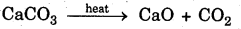Question 10.
Why is respiration considered an exothermic reaction ? Explain.
In respiration, food is broken or oxidised in the presence of oxygen inhaled. In this process, energy is liberated. So respiration is called an exothermic reaction.
C2H12O6 + 6O2 → 6CO2 + 6H2O + Energy

Question 11.
Why are decomposition reactions called the opposite of combination reactions ? Write equations for these reaction.
Decomposition reactions are those reactions in which a compound is broken into two Or more new compounds.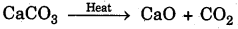Combination reactions are those reactions in which two substances are combined to form a new substance.
CaO + CO2 → CaCO3
In above examples, both the reactions are same but show opposite directions; so decomposition reaction is called the opposite of combination reaction.Question 12.
Write one equation each for decomposition reactions where energy is supplied in the form of heat, light or electricity.
Energy supplied, in the form of heat :Energy supplied in the form of light :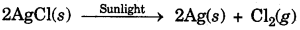Energy supplied in the form of electricity :Question 13.
What is the difference between displacement and double displacement reactions ? Write equations for these reactions.
In displacement reaction, one element from its salt is displaced by a more reactive element. For example in following reaction, Cu is displaced by Zn from CuSO4 because Zn is more reactive.
CuSO4 + Zn → ZnSO4 + Cu
In double displacement reaction, exchange of ions takes place between two reactants to forms new products.
Na2SO4(aq) + BaCl2(aq) → BaSO4(s) + 2NaCl(aq)

Question 14.
In the refining of silver, the recovery of silver from silver nitrate solution involves displacement by copper metal. Write down the reaction involved.
When copper is mixed in silver nitrate solution, it displaces the silver becaiise copper is more reactive than silver.
2AgNO3+ Cu → CU(NO3)2 + Ag

Question 15.
What do you mean by a precipitation reaction ? Explain giving examples.
The reactions in which a precipitate is formed are called precipitation reactions.
Examples :
1. Na2SO4(aq) + BaCl2(aq) → BaSO3(s) + 2NaCl(ag) (Precipitate)
2. Pb(NO3)2 + 2KI → 2KNO3 + PbI2

Question 16.
Explain the following terms of gain or loss of oxygen with two examples each :
(a) Oxidation
(b) Reduction .
(a) Oxidation : The reactions in which gain of oxygen takes place are called oxidation.
Examples :
1. 2Cu + O2→ 2CuO
2. 2H2 + O2 → 2H2O

(b) Reduction : The reactions in which loss of oxygen takes place are called reduction.
Examples :Question 17.
A shiny brown coloured element X on heating in air becomes black in colour. Name the element X and black coloured compound formed.
The shiny brown coloured element X is copper. When it is heated in air, it becomes black due to the deposit of copper oxide.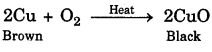Question 18.
Why do we apply paint on iron articles ?
We apply paint on iron, articles to prevent them from corrosion. Paint disconnects the relation between iron and air or water.

Question 19.
Oil and fat containing food items are flushed with nitrogen. Why?
Oil and fat containing food items are flushed with nitrogen to prevent them from damage. When such items come in contact of air, they get oxidised and become rancid. Their smell and taste change.Question 20.
Explain the following terms with an example each :
(a) Corrosion
(b) Rancidity
(a) Corrosion : The process of damaging or chemically eaten up the surface of some metals when they are left for some time in moist air is called corrosion.

Conditions of corrosion :
1. Presence of moisture (water)
2. Presence of air
Example : Rusting of iron is a common example of corrosion.

(b) Rancidity : When fats and oils are oxidised, they become rancid and their smell and taste change. This phenomenon is called rancidity.
Example : Fat and oil containing substances are damaged and their taste or smell is changed.

### Bihar Board Class 10 Science Chapter 1 Chemical Reactions and Equations Textbook Activities

Activity 1.1 (Page 1)

CAUTION : This activity needs teacher’s assistance. it would be better if students wear eye protection.

• Clean a magnesium ribbon, about 2 cm long, by rubbing it with sand paper.
• Hold it with a pair of tongs. Burn it using a spirit lamp or a burner and collect the ash so formed in a watch glass as shown in Fig. 1.1. Burn a magnesium ribbon keeping it as far as possible from your eyes.Question 1.
What do you observe?
We observe that magnesium ribbon burns with a dazzling white flame and changes into a white powder. This powder is magnesium oxide. Ìt s formed due to the reaction between magnesium and oxygen present in the air.
2Mg + O2 → 2MgO

Activity 1.2 (Page 2)

• Take ld nitrate solution in a test tube.
• Add potšium iodide solution to this.

Question 1.
What do you observe?
We observe that both the substances react together and the following reaction takes place :
Pb(NO3)2 + 2KI → 2KNO3 + PBI2

Activity 1.3 (Page 2)

• Take a few zinc granules in a conical flask or a test tube.
• Add dilute hydrochloric acid or sulphuric acid to this (Fig. 1.2)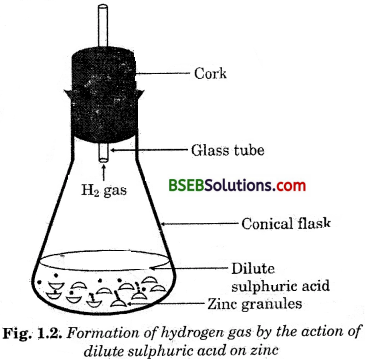CAUTION : Handle the acid with care.

Question 1.
Do you observe anything happening around the zinc granules?
Yes, zinc granules start tó decrease in size and hydrogenQuestion 2.
Touch the conical flašk or test tube. Is there any change in its temperature?
Yes, its temperature is slightly increased.
Note : From the above three activities, we can conclude that following observations that help us to determine whether a chemical reaction has taken place :

1. change in state
2. change in colour
3. evolution of gas
4. change in temperature.

Activity 1.4 (Page 6)

• Take a small amount of calcium oxide or quick lime in a beaker.
• Slowly add water to this.
• Touch the beaker as shown in Fig. 1.4.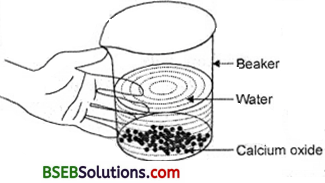Fig. 1.4 Formation of šlaked lime by the reaction of calcium oxide with water

Question 1.
Do you feel any change in temperature?
Yes, its temperature has increased highly. The following reaction takes place :
CaO + H2O → Ca(OH)2+ Heat

Activity 1.5 (Page 8)

• take about 2 g ferrous sulphate crystals in a dry boiling tube.
• Note the colour of the ferrous sulphate crystals.
• Heat the boiling tube over the flame of a burner or spirit lamp as shown in Fig. 1.5.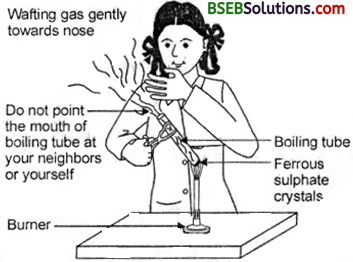Fig. 1.5. Correct way of heating the test tube containing crystals of ferrous sulphate and of smelling the odour

Question 1.
Observe the colour of the crystals after heating. Ans. Observation: The colour of ferrous sulphate crystals is green before heating. It becomes colourless after heating.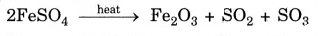Activity 1.6 (Page 8)

• Take about 2 g lead nitrate powder in a boiling tube.
• Hold the boiling tube with a pair of tongs and heat it over na flame as shown in Fig. 1.6.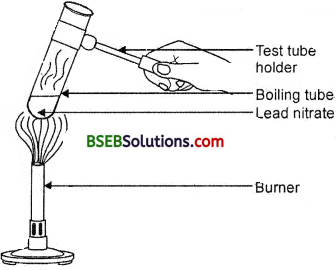Fig. 1.6. Heating of lead nitrate and emission of nitrogen dioxide

Question 1.
What do you i observe ? Note the change, if any. Ans. Observation: We observe the emission of brown fumes. These fumes are of nitrogen dioxide (NQ2). The following reaction takes place :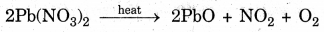Activity 1.7 (Page 9)

• Take a plastic mug. Drill two holes at its base and fit rubber stopper in these holes. Insert carbon electrodes in these rubber stoppers as shown in Fig. 1.7.
• Connect these electrodes to a 6 volt battery.
• Fill the mug with water such that the electrodes are immersed. Add a few drops of dilute sulphuric acid to the water.
• Take two test tubes filled with water and invert them over two carbon electrodes.
• Switch on the current and leave the apparatus undisturbed * for some time.
• You will observe the formation of bubbles at both the electrodes. These bubbles displace water in the test tubes.

Question 1.
Is the volume of the gas collected the same in both the test tubes ?

• No, volume of gas in both the test tubes is not the same. Once the test tubes are filled with the respective gases, remove them carefully.
• Test these gases one by one by bringing a burning candle close to the mouth of the test tubes.

CAUTION : This step must be performed carefully by the teacher.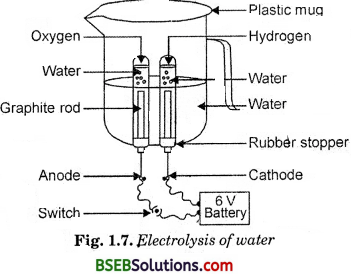Question 2.
What happens in each case ?
The volume of gas in one test tube is twice the volume of gas in the other test tube.

Question 3.
Which gas is present in each test tube ?
In one test tube, hydrogen gas is present, while oxygen js present in the other test tube.Activity 1.8 (Page 9)

• Take about 2 g silver chloride on a china dish.

Question 1.
What is the colour of silver chloride ?
It is white coloured.
Place this china dish under sunlight for some time (Fig. 1.8).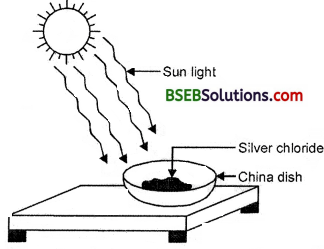Fig. 1.8 Silver chloride turns grey in sunlight to form silver metal

Question 1.
Observe the colour of the silver chloride after some time.
Observation : The white colour of silver chloride turns grey in sunlight. It is due to decomposition of silver chloride into silver and chlorine by light.Activity 1.9 (Page 10)

• Take three iron nails and clean them by rubbing with sand paper.
• • Take two test tubes marked as.(A) and (B). In each test tube, take about 10 ml copper sulphate solution.
• • Tie two iron nails with a thread and immerse them carefully in the copper sulphate solution in test tube B for about 20 minutes [Fig. 1.9 (a)]. Keep one iron naif aside for comparison.
• • After 20 minutes, take out the iron nails from the copper ‘ sulphate solution.
• Compare the intensity of the blue colour of copper sulphate solutions in test tubes (A) and (B) [Fig. 1.9 (b)].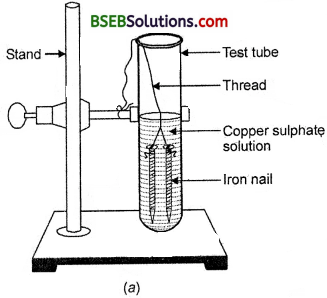(a) Iron nails dipped in copper sulphate solution
(b) Iron nails and copper sulphate solutions cornpared before and after the experi?ment

• Also, .compare the colour of the iron nails dipped in the copper sulphate solution with the one kept aside [Fig. 1.9 (6)].

Questions Based on the Activity
Question 1.
What is the colour of copper sulphate solution in test tube A?
Blue colour.

Question 2.
What is the colour of copper sulphate solution in test tube B ?
The blue colour in test-tube B is faded.

Question 3.
Why does the blue colour of copper sulphate solution fade ?
The colour of copper sulphate solution fades due to displacement of copper.Question 4.
What is the colour of iron nail in test tube B ?
Its colour becomes brown.

Question 5.
Why does the iron nail become brownish in colour ?
The colour of iron nail becomes brown due to deposition of copper over iron nail.
CuS04 + Fe → FeS04 + Gu

Activity 1.10 (Page 11)

• Take about 3 ml of sodium sulphate solution in a test tube.
• In another test tube, take about 3 ml of barium chloride solution.
• Mix the two solutions (Fig. 1.10).Question
What do you observe ?
We observe that a white substance is formed which is insoluble in water.
Na2S04 + BaCl2 → 2NaCl + BaS04

Activity 1.11 (Page 12)

• Heat a china dish containing about 1 g copper powder (Fig. 1.11).Question
What do you observe ?
We observe that the surface of copper powder becomes coated with black copper (II) oxide. It is formed by the reaction of copper powder with oxygen.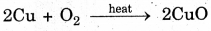### Bihar Board Class 10 Science Chapter 1 Chemical Reactions and Equations NCERT Exemplar Problems

Question 1.
Write the balanced chemical equations for the following reactions and identify the /type of reaction in each case.
(a) Nitrogen gas is treated with hydrogen gas in the presence of a catalyst at 773 K to form ammonia gas.
(b) Sodium hydroxide solution is treated with acetic acid to form sodium acetate and water.
(c) Ethanol is warmed with ethanoic acid to form ethyl acetate in the presence of concentrated HgSO4.
(d) Ethene is burnt in the presence of oxygen to form carbon dioxide, water and releases heat and light.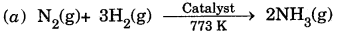Combination reaction.

(b) NaOH(aq) + CH3COOH(aq) → CH3COONa(aq) + H2O(l)
Double displacement reaction / Neutralisation reaction.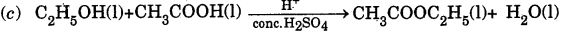Double displacement reaction/Esterificaton reaction.

(d) C2H4(g) + 3O2(g) → 2CO2(g) + 2H2O(g) + Heat + Light
Redox reaction/Combustion reaction.

Question 2.
Write the balanced chemical equations for the following reactions and identify the type of reaction in each case.
(а) Thermit reaction, iron (III) oxide reacts with aluminium and gives molten iron and aluminium oxide.
(b) Magnesium ribbon is burnt in an atmosphere of nitrogen gas to form solid magnesium nitride.
(c) Chlorine gas is passed in an aqueous potassium iodide solution to form potassium chloride solution and solid iodine.
(d) Ethanol is burnt in air to form carbon dioxide, water and releases heat.
(a) Fe2O3(s) + 2Al(s) → Al2O3(s) + 2Fe(l) + Heat
Displacement reaction/Redox reaction.
(b) 3Mg(s) + N2(g) → Mg3N2(s) Combination reaction.
(c) 2KI(aq) +Cl2(g) → 2KCl(aq) + I2(s) Displacement reaction.
(d) C2H5OH(l) + 3O2(g) → 2CO2(g) + 3H2O(l) + Heat Redox reaction/Gombustion reaction.

Question 3.
Complete.the missing components/variables given as x and y in the following reactions :
(а) Pb(NO3)2(aq) + 2KI(aq) → PbI2(x) + 2KNO3(y)
(b) Cu(s) + 2AgNO3(aq) → Cu(NO3)2(aq) + x(s)
(c) Zn(s) + H2SO4(aq) → ZnSO4(x) + H2(y)(a) x → (s) y → (aq)
(b) x → 2Ag
(c) x → (aq) y → (g)
(d) x → Heat

Question 4.
Which among the following changes are exothermic or endo-thermic in nature?
(а) Decomposition of ferrous sulphate.
(b) Dilution of sulphuric acid.
(c) Dissolution of sodium hydroxide in water.
(d) Dissolution of ammonium chloride in water.
(b) and (c) are exothermic as heat is released in these changes.
(a) and (d) are endothermic as heat is absorbed in these changes.

Question 5.
Identify the reducing agent in the following reactions
(а) 4NH3 + 5O2 → 4NO + 6H2O
(b) H2O + F2 → HF + HOF
(c) Fe2O3 + 3CO → 2Fe + 3CO2
(d) 2H2 + O2 → 2H3O
Reducing agents are those substances which have the ability of adding hydrogen or removing oxygen from the other substances.
(a) Ammonia (NH3)
(b) Water (H2O)
(c) Carbon monoxide (CO)
(d) HydrogenQuestion 6.
Identify the oxidising agent (oxidant) in the following reactions :
(a) Pb3O4 + 8HCl → SPbClg + Cl2 + 4H2O
(b) 2Mg + O2 → 2MgO
(c) CuSO4 + Zn → Cu + ZnSO4
(d) V2O5 + 5Ca → 2V + 5CaO
(e) 3Fe + 4H2O → Fe3O4 + 4H2
(f) CuO + H2 → Cu + H2O
(a) Pb3O4
(b) O2
(c) CuSO4
(d) V2O5
(e) H2O
(f) CuO

Question 7.
Write the balanced chemical equations for the following reactions :
(a) Sodium carbonate on reaction with hydrochloric acid in equal molar concentrations gives sodium chloride and sodium hydrogencarbonate.
(b) Sodium hydrogencarbonate on reaction with hydrochloric acid gives sodium chloride, water and liberates carbon dioxide.
(c) Copper sulphate on treatment with potassium iodide precipitates cuprous iodide (Cu2I2), liberates iodine gas and also forms potassium sulphate.
(a) Na2CO3 + HCl → NaCl + NaHCO3
(b) NaHCO3 + HCl →NaCl + H2O + CO2
(c) 2CuSO4 + 4KI → Cu2I2 + 2K2SO4 + I2

Question 8.
A solution of potassium chloride when mixed with silver nitrate solution, an insoluble white substance is formed. Write the chemical reaction involved and also mention the type of the chemical reaction?
KCl(aq) + AgNO3(aq) → AgCl(s) + KNO3(aq)
It is a double displacement and precipitation reaction.

Question 9.
Ferrous sulphate decomposes with the evolution of a gas having a characteristic odour of burning sulphur. Write the chemical reaction involved and identify the type of reaction.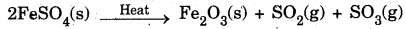It is a thermal decomposition reaction.Question 10.
Why do fire flies glow at night?
Fire flies have a protein which in the presence of an enzyme undergoes aerial oxidation. This is a chemical reaction which involves emission of visible light. Therefore, fire flies glow at night.

Question 11.
Grapes hanging on the plant do not ferment but after being plucked from the plant can be fermented. Under what conditions do these grapes ferment? Is it a chemical or a physical change?
Grapes when attached to the plants are living and therefore their own immune system prevents fermentation. The microbes can grow in the plucked grapes and under anaerobic conditions these can be fermented. This is a chemical change.

Question 12.
Which among the following are physical or chemical changes?
(а) Evaporation of petrol.
(b) Burning of Liquefied Petroleum Gas (LPG).
(c) Heating of an iron rod to red hot.
(d) Curdling of milk.
(e) Sublimation of solid ammonium chloride.
Physical changes : The processes in which no new substances are formed are called physical changes.
Chemical changes : The processes in which new substances are formed are called chemical changes.
So, it is clear that :
(a), (c) and (e) are physical changes
(b) and (d) are chemical changes.

Question 13.
During the reaction of some metals with dilute hydrochloric acid, following observations were made :
(a) Silver metal does not show any change.
(b) The temperature of the reaction mixture rises when aluminium (Al) is added.
(c) The reaction of sodium metal is found to be highly explosive.
(d) Some bubbles of a gas are seen when lead (Pb) is treated with the acid.
Explain these observations giving suitable reasons.
(a) Silver metal does not show any change because it does not react with dilute HCl.
(b) The temperature of the reaction mixture rises when aluminium is added because it is an exothermic reaction.
(c) Reaction of sodium metal is found to be highly explosive because it is an exothermic reaction.
(d) When lead is treated with hydrochloric acid, bubbles of hvdrogen gas are evolved.
Pb + 2HCl → PbCl2 + H2Question 14.
A substance X, which is an oxide of a group 2 element, is used intensively in the cement industry. This element is present in bones also. On treatment with water it forms a solution which turns red litmus blue. Identify X and also write the chemical reactions involved.
The substance which is an oxide of a group 2 element used intensively in the cement industry and on treatment with water forms a solution which turns red litmus blue is ‘calcium oxide’. The chemical reactions involved in the treatment of calcium oxide with water is as follows :
CaO(s) + H2O(l) → Ca(OH)2(aq)

Question 15.
Write a balanced chemical equation for each of the following reactions and also classify them :
(а) Lead acetate solution is treated with dilute hydrochloric acid to form lead chloride and acetic acid solution. ,
(b) A piece of sodium metal is added to absolute ethanol to form sodium ethoxide and hydrogen gas.
(c) Iron (III) oxide bn heating with carbon monoxide gas reacts to form solid iron and liberates carbon dioxide gas.
(d) Hydrogen sulphide gas reacts with oxygen gas to form solid sulphur and liquid water.
(a) Pb(CH3COO)2(l) + 2HCl(aq) → PbCl2(l) + CH3COOH(l)
Double displacement reaction.
(b) 2Na + 2C2H5OH → 2C2H5ONa + H2
Displacement reaction.
(c) Fe2O2 + 3CO(g) → 2Fe(s) + 3CO2(g) Redox reaction.
(d) 2H2S(g) + O2(g) → 2S(s) + 2H2O(l) Redox reaction.

Question 16.
Why do we store silver chloride in dark coloured bottles?
Silver chloride on exposure to sunlight may decompose as per the following reaction :
2AgCl → 2Ag + Cl2
Therefore, it is stored in dark coloured bottles.

Question 17.
Balance the following chemical equations and identify the type of chemical reaction :
(a) Mg(s) + Cl2(g) → MgCl2(s)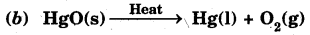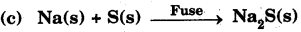(d) TiCl2(l) + Mg(s) → Ti(s) + MgCl2(s)
(e) CaO(s) + SiO2(s) → CaSiO3(s)(a) Balanced; Combination reaction.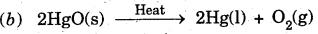Decomposition reaction.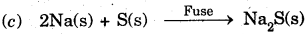Combination reaction.

(d) TiCl4(l) + 2Mg(s) → Ti(s) + 2MgCl2(s)
Displacement reaction.

(e) Balanced; Combination reaction.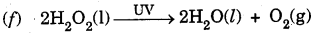Decomposition reaction.Question 18.
A magnasium ribbon is burnt in oxygen to give a white compound X accompanied by emission of light. If the burning ribbon is now placed in an atmosphere of nitrogen, it continues to burn and forms a compound Y.
(а) Write the chemical formulae of X and Y.
(b) Write a balanced chemical equation, when X is dissolved in water.
(a) When a magnesium ribbon is burnt in oxygen, magnesium oxide (a white compound) is formed accompanied by the emission of light.
2Mg + O2 → 2MgO
Therefore, the chemical formula of X is MgO. If the burning ribbon is placed in an atmosphere of nitrogen, it continues to burn and forms magnesium nitride.
3Mg + N2 → Mg3N2
Therefore, the chemical formula of Y is Mg3N2.

(b) When X i.e., MgO is dissolved in water, magnesium hydroxide is formed. The balanced chemical equation for this reaction is as follows :
MgO + H2O → Mg(OH)2

Question 19.
Zitic liberates hydrogen gas when reacted with dilute hydrochloric acid, whereas copper does not. Explain why?
Zinc is above hydrogen whereas copper is below hydrogen in the activity series of metals. That is why zinc displaces hydrogen from dilute hydrochloric acid, while copper does not.
Zn + 2HCl → ZnCl2 + H2
Cu + HCl → No reaction

Question 20.
A silver article generally turns black when kept in the open for a few days. The article when rubbed with toothpaste again starts shining.
(a) Why do silver articles turn black when kept in the open for a few days? Name the phenomenon involved.
(b) Name the black substance formed and give its chemical formula.
(a) Silver articles turn black when kept in the open for a few days because metals such as silver when attacked by substances around it such as gases, moisture, acids, etc. get corrode and this phenomenon is called corrosion.
(b) The black substance is formed because silver (Ag) reacts with H2S present in air. It forms thin black coating of silver sulphide (Ag2S)Question 21.
On heating blue coloured powder of copper (II) nitrate in a boiling tube, copper oxide (black), oxygen gas and a brown gas X is formed
(a) Write a balanced chemical equation of the reaction.
(b) Identify the brown gas X evolved.
(c) Identify the type of reaction.
(d) What could be the pH range of aqueous solution of the gas X ?
(a) Balanced chemical equation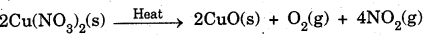(b) The brown gas X evolved is nitrogen dioxide (NO2 ).
(c) This is a decomposition reaction.
(d) Nitrogen dioxide dissolves in water to form acidic solution because it is an oxide of non-metal. Therefore, pH of this solution is less than 7.

Question 22.
Give the characteristic tests for the following gases :
(a) CO2
(6) SO2
(c) O2
(d) H2
(a) The characteristic test for carbon dioxide (CO2 ) gas turns lime water milky when passed through it due to the formation of insoluble calcium carbonate.(b) Sulphur dioxide (SO2 ) gas when passed through acidic potassium permanganate solution (purple in colour) turns it colourless because SO2 is a strong reducing agentor
Sulphur dioxide gas when passed through acidic dichromate solution (orange in colour) turns it to green because sulphur dioxide is a strong reducing agent.

(c) The evolution of oxygen (O2 ) gas during a reaction can be confirmed by bringing a burning candle near the mouth of the test tube containing the reaction mixture. The intensity of the flame increases because oxygen supports burning.
(d) Hydrogen (H2 ) gas burns with a pop sound when a burning candle is brought near it.Question 23.
What happens when a piece of –
(а) zinc metal is added to copper sulphate solution?
(b) aluminium metal is added to dilute hydrochloric acid?
(c) silver metal is added to copper sulphate solution?
Also, write the balanced chemical equation if the reaction occurs.
(a) When zinc metal is added to copper sulphate solution zinc being more reactive than copper displaces copper from its solution and a solution of zinc sulphate is obtained.This is an example of displacement reaction.

(b) When aluminium metal is added to dilute hydrochloride acid, aluminium being more reactive displaces hydrogen from dilute hydrochloric acid solution and hydrogen gas is evolved.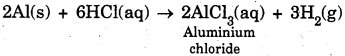(c) When silver metal is added to copper sulphate solution no reaction occurs because silver metal being less reactive than copper cannot displace copper from its salt solution.
Ag(s) + CuSO4(aq) → No reactionQuestion 24.
What happens when zinc granules are treated with dilute solution of H2SO4, HCl, HNO3, NaCl and NaOH. Also write the chemical equations if reaction occurs.
When zinc granules are treated with –

(a) dilute solution of H2SO4, zinc sulphate (ZnSO4) is formed and hydrogen gas is evolved.
Zn(s) + H2SO4(aq) → ZnSO4(aq) + H2(g)

(b) dilute solution of HCl, zinc chloride (ZnCl2) is formed and hydrogen gas is evolved.
Zn(s) + 2HCl(aq) → ZnCl2(aq) + H2(g)

(c) dilute solution of HNO3, zinc nitrate, water and nitrous oxide is formed.
4Zn(s) + lOHNO3(aq) → 4Zn(NO3)2(aq) + 5H2O(l) + N2O(g)
(Reaction with dilute HNO3 is different as compared to other acids because nitric acid is an oxidising agent and it oxidises H2 gas, so N2O is evolved).

(d) dilute NaCl solution, no reaction occurs.
Zn(s) + NaCl(aq) → No reaction

(e) dilute NaOH solution, sodium zincate and hydrogen gas are formed.Question 25.
On adding a drop of barium chloride solution to an aqueous solution of sodium sulphite, white precipitate is obtained,
(a) Write a balanced chemical equation of the reaction involved
(b) What other name can be given to this precipitation reaction?
(c) On adding dilute hydrochloric acid to the reaction mixture, white precipitate disappears. Why?
(a) Balanced chemical equation for the reaction that occurs when a drop of barium chloride solution is added to an aqueous solution of sodium sulphite :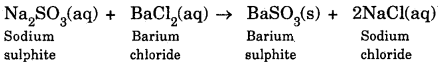(b) This reaction is also known as double displacement reaction.
(c) BaSO3 is a salt of a weak acid (H2SO3), therefore dilute acid such as HCl decomposes barium sulphite to produce sulphur dioxide gas which has the smell of burning sulphur.
BaSO3(s)(White ppt) + 2HCl(aq) BaCl2 + H2O + SO2(g)
BaCl2 is soluble in water, hence white precipitate disappears.Question 26.
You are provided with two containers made up of copper and aluminium. You are also provided with  solutions of dilute HCl, dilute HNO3, ZnCl2 and H2O. In which of the above containers these solutions can be kept ?
(A) When solutions are kept in copper container
(а) Dilute HCl
Copper does not react with dilute HCl. Therefore, it can be kept.

(b) Dilute HNO3
Nitric acid acts as a strong oxidising agent ana reacts with copper vessel, therefore cannot be kept.

(c) ZnCl2
Zinc is more reactive than copper (Cu) therefore, no  displacement reaction occurs and hence can be kept.

(d) H3O
Copper does not react with water. Therefore, can be kept.

(B) When solutions are kept in aluminium containers
(a) Dilute HCl
Aluminium reacts with dilute HCl to form its salt and hydrogen is evolved. Therefore, cannot be kept.
2Al + 6HCl → 2AICl3 + 3H2(b) Dilute HNO3
Aluminium gets oxidised by dilute HNOg to form a layer of A1203 and can be kept.

(c) ZnCl2
Aluminium being more reactive than zinc can displace zinc ion from the solution. Therefore, the solution cannot be kept.
2Al + 3ZnCl2 → 2AlCl3 + 3Zn

(d) H2O
Aluminium does not react with cold or hot water. Therefore, water can be kept.
Aluminium is attacked by steam to form aluminium oxide and hydrogen
2Al (s) + 3H2O (g) → Al2O3(S) + 3H2(g)